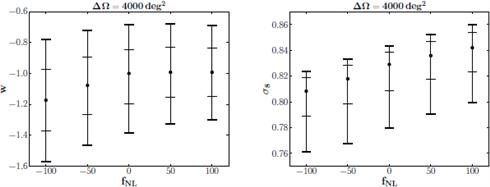CAUP Researchers: Arlindo M. M. Trindade, Pedro P. Avelino, Pedro T. P. VianaTeam at CAUP: Galaxies and Observational CosmologyBiased cosmological parameter estimation with galaxy cluster counts in the presence of primordial non-Gaussianities,Monthly Notices of the Royal Astronomical Society, Volume 435, pp. 782 (2013)The redshift dependence of the abundance of galaxy clusters is very sensitive to the statistical properties of primordial density perturbations. It can thus be used to probe small deviations from Gaussian initial conditions. Such deviations constitute a very important signature of many inflationary scenarios, and are thus expected to provide crucial information on physical processes which took place in the very early Universe. We have determined the biases which may be introduced in the estimation of cosmological parameters by wrongly assuming the absence of primordial non-Gaussianities. Although we find that the estimation of the present-day dark energy density using cluster counts is not very sensitive to the non-Gaussian properties of the density field, we show that the biases can be considerably larger in the estimation of the dark energy equation of state parameter w and of the amplitude of the primordial density perturbations. Our results suggest that a significant level of non-Gaussianity at cluster scales may be able to reconcile the constraint on the amplitude of the primordial perturbations obtained using galaxy cluster number counts from the Planck Sunyaev-Zeldovich Catalog with that obtained from the primary cosmic microwave background anisotropies measured by the Planck satellite.Figure 1 | The most probable values of the dark energy equation of state parameter, w, and the present-day root mean square mass perturbation, σ8, and associated 1σ (inner thin ticks) and 2σ (outer thick ticks) confidence levels, for a sky coverage of 4000 deg2. These parameters are represented as a function of the level of non-gaussianity, fNL.For many years, inflation has been the most widely accepted model to explain the density perturbations observed in the universe and the most simple inflation models consider that the initial density perturbations presented a Gaussian distribution. This assumption seems to be in overall agreement with current observational data but many inflationary models present, instead, a certain degree of non-gaussianity, with potentially observable consequences for this different distribution. As a way to narrow down the list of inflation models compatible with the observational data, it is mandatory to determine which would be the observable consequences of different levels of non-gaussianity in the initial density perturbations. In order to achieve this, three major observational quantities were considered: the present-day dark energy density, the dark energy equation of state parameter and the present-day root mean square mass perturbations at the 8 h-1 Mpc scale. These quantities were estimated for 5 different levels of non-gaussianity assuming a flat ΛCDM fiducial cosmological model and an observed sky area of 4000 deg2. With these parameters, it was estimated how many galaxy clusters were expected to be observed in a given range of redshift and mass, with a lower limit of 5x1014 h-1 M☉. The bias arising in the estimation of the observable quantities if the density perturbations were wrongly assumed to be Gaussian could then be calculated for each level of non-gaussianity from the obtained distributions of galaxy clusters. The results obtained show a modest bias arising in the present-day dark energy density and in the dark energy equation of state parameter from considering Gaussian perturbations in the case they had, in fact, a level of non-gaussianity. However, for the present-day root mean square mass perturbations, the bias is significantly larger (Fig. 1). Even so, excluding the fiducial value for this quantity with 2σ significance would require a level of non-gaussianity of about -80 or significantly above 100. Although the results obtained from the Planck satellite data ensure the local level of non-gaussianity is very small (2.7±5.8), this could be a function of scale and there is actually some evidence that at cluster scales this parameter may have a value of about -240. Thus, this work demonstrates it is important to consider the effect of non-gaussianity in the estimation of the present-day root mean square mass perturbations.

# Institute of Astrophysics and Space Sciences

Institute of Astrophysics and Space Sciences (IA) is a new but long anticipated research infrastructure with a national dimension. It embodies a bold but feasible vision for the development of Astronomy, Astrophysics and Space Sciences in Portugal, taking full advantage and fully realizing the potential created by the national membership of the European Space Agency (ESA) and the European Southern Observatory (ESO). IA resulted from the merging the two most prominent research units in the field in Portugal: the Centre for Astrophysics of the University of Porto (CAUP) and the Center for Astronomy and Astrophysics of the University of Lisbon (CAAUL). It currently hosts more than two-thirds of all active researchers working in Space Sciences in Portugal, and is responsible for an even greater fraction of the national productivity in international ISI journals in the area of Space Sciences. This is the scientific area with the highest relative impact factor (1.65 times above the international average) and the field with the highest average number of citations per article for Portugal.

 Proceed on CAUP's website | Go to IA website